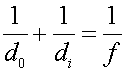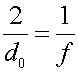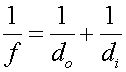Examples for mirrors

Example #1

Problem:

a.) An image is located at exactly the same position as its object, for a mirror of focal length 6 cm. What is the object distance?

Solution:

Use the formulawith di and do equal to getdo = 12 cm

b.) If the height of the object is 4.5 mm, what is the height of the image?

Solution:

Useto see that the image height equals the object height.

hi = - 4.5 mm (inverted)

Example #2

Problem:

a.) A convex mirror has an object 14 cm from the mirror, and the image appears to be 7 cm behind the mirror. What is the focal length of the mirror?

Solution:

Use the formulawith the image distance negative.

f = -14 cm

b.) If the object height is 8.0 mm, what is the height of the image?

Solution:

Use the formulawith the image distance negative.

hi = 4.0 cm (upright)

Example #3

Problem:

Suppose one wishes to use a mirror as a projector, to illuminate a small object of height 1.0 cm, and display the image on a screen at a size of 1.0 m where the distance to the screen is 4.0 m.

a.) What kind of mirror should one use? (concave or convex)

concave

b.) What should the focal length of the mirror be?

Solution: First calculate the object distance via the magnification formula. One obtains: do = 4.0 cm. Next, calculate the focal length via. Since di is large compared to do, the focal length will be approximately equal to do.

f = 4.0 cm

Mirrors index        Lecture index